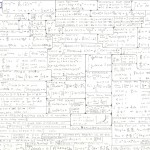# Math notes for entry-level grad studentsThis post points to a handwritten one-page summary of mathematics, at a level expected of first-year grad students specializing in engineering mechanics: http://csm.mech.utah.edu/content/wp-content/uploads/2012/05/NotesMath.jpg

# Tutorial: Functional and Structured Tensor Analysis for Engineers

A step-by-step introduction to tensor analysis that assumes you know nothing but basic calculus. Considerable emphasis is placed on a notation style that works well for applications in materials modeling, but other notation styles are also reviewed to help you better decipher the literature. Topics include: matrix and vector analysis, properties of tensors (such as “orthogonal”, “diagonalizable”, etc.), dyads and outer products, axial vectors, axial tensors, scalar invariants and spectral analysis (eigenvalues/eigenvectors), geometry (e.g., the equations for planes, ellipsoids, etc.), material symmetry such as transverse isotropy, polar decomposition, and vector/tensor calculus theorems such as the divergence theorem and Stokes theorem. (A draft of this document was last released publically on Aug. 3, 2003. The non-public version is significantly expanded in anticipation of formal publication.)